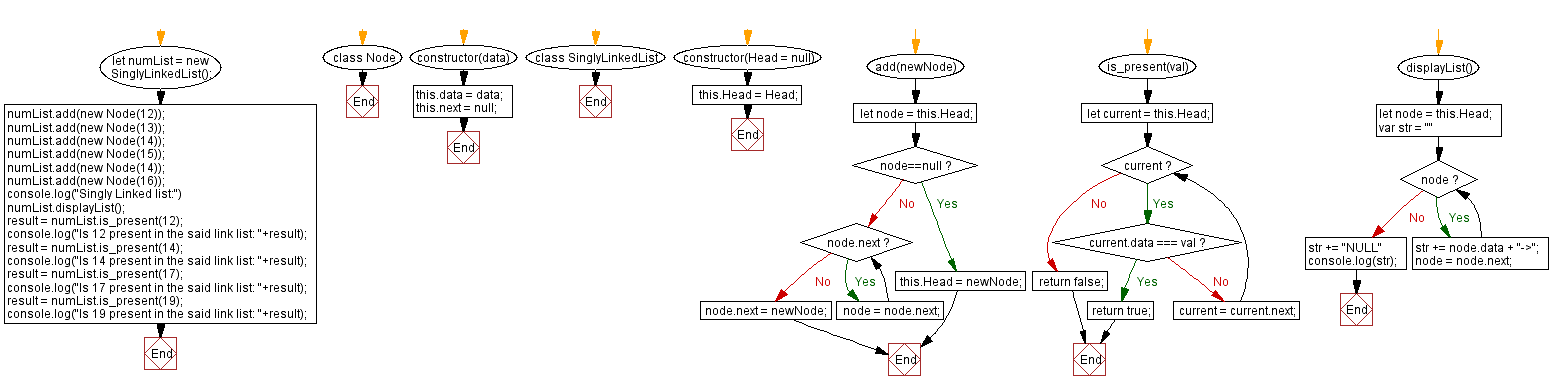# JavaScript Exercises: Check if an element is present in the Singly Linked list

## JavaScript Singly Linked List: Exercise-18 with Solution

Write a JavaScript program to check if an element is present in the Singly Linked list.

Sample Solution:

JavaScript Code:

``````class Node {
constructor(data) {
this.data = data;
this.next = null;
}
}
}

if(node==null){
return;
}
while (node.next) {
node = node.next;
}
node.next = newNode;
}

is_present(val) {
while (current) {
if (current.data === val) {
return true;
}
current = current.next;
}
return false;
}

displayList(){
var str = ""
while (node) {
str += node.data + "->";
node = node.next;
}
str += "NULL"
console.log(str);
}
}
numList.displayList();
result = numList.is_present(12);
console.log("Is 12 present in the said link list: "+result);
result = numList.is_present(14);
console.log("Is 14 present in the said link list: "+result);
result = numList.is_present(17);
console.log("Is 17 present in the said link list: "+result);
result = numList.is_present(19);
console.log("Is 19 present in the said link list: "+result);
```
```

Sample Output:

```Singly Linked list:
12->13->14->15->14->16->NULL
Is 12 present in the said link list: true
Is 14 present in the said link list: true
Is 17 present in the said link list: false
Is 19 present in the said link list: false
```

Flowchart:Live Demo:

See the Pen javascript-singly-linked-list-exercise-18 by w3resource (@w3resource) on CodePen.

Improve this sample solution and post your code through Disqus

Singly Linked List Previous: Get index of an given element in a Singly Linked list.

What is the difficulty level of this exercise?

Test your Programming skills with w3resource's quiz.

﻿

## JavaScript: Tips of the Day

Returns the average of an array, after mapping each element to a value using the provided function

Example:

```const averageBy = (arr, fn) =>
arr.map(typeof fn === 'function' ? fn : val => val[fn]).reduce((acc, val) => acc + val, 0) /
arr.length;

console.log(averageBy([{ n: 2 }, { n: 4 }, { n: 6 }, { n: 8 }], o => o.n));
console.log(averageBy([{ n: 1 }, { n: 3 }, { n: 5 }, { n: 7 }], 'n'));
```

Output:

```5
4
```